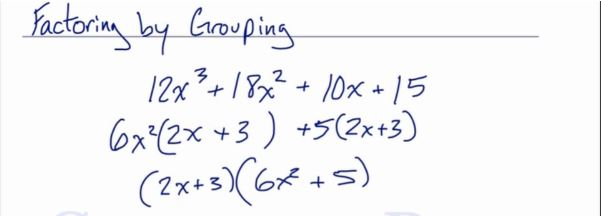In this video, we are going to look at how to factor by grouping. After you finish this lesson, view all of our Algebra 1 lessons and practice problems.

For example:
To factor$12x^3+18x^2+10x+15$, first think about it in two pieces, cutting it in half. Then we will look at the first two terms, and take out the greatest common factor, which is$6x^2$. This will leave us with$6x^2(2x+3)$
Then, we do the same thing for the next two terms. The greatest common factor here is 5. When we take out the greatest common factor, we are left with$5(2x+3)$
Notice that the same exact factors are written in both sets of parentheses. From here we can factor out the$(2x+3)$ from each term. This will result in a final answer of$(2x+3)(6x^2+5)$Examples of Factoring by Grouping

Example 1

Factor$x^3+7x^2+2x+14$

Group the first two terms together and then the last two terms together.$(x^3+7x^2)+(2x+14)$
Factor out a GCF from each separate binomial.
x^2(x+7)+x^2(x+7)
Factor out the common binomial.
(x+7)(x^2+2)

Example 2

Factor$3x^2+xy-12x-4y$
Group the first two terms together and then the last two terms together.$(3x^2+xy)-(12x-4y)$
Factor out a GCF from each separate binomial.$x(3x+y)-4(3x-y)$
Factor out the common binomial.$(3x-y)(x-4)$

Video-Lesson Transcript

Let’s go over factoring by grouping.

We have$12x^3 + 18x^2 + 10x + 15$

So we’re going to factor this four-term polynomial by grouping.

We’re going to split this in half.

Let’s look at the first two terms of the polynomial.$12x^3 + 18x^2$

And we’ll find out what is the greatest common factor of these two terms.

It’s$6x^2$.

Let’s factor.$12x^3 + 18x^2$$6x^2 (2x + 3)$

Now let’s do the same with the other two terms.$10x + 15$

The greatest common factor for these two is$5$.

Let’s factor.$10x + 15$$+5 (2x + 3)$

Let’s put the plus sign.

At this point, we have the same exact factors:$2x + 3$

We can actually factor this out of both of these terms.

We have$6x^2 (2x + 3) +5 (2x + 3)$

So let’s take the same factors out.$(2x + 3) (6x^2 + 5)$

Let’s take a look at another example.$6x^3 + 7x^2 - 42x - 49$

Again, let’s split the polynomial into two.

Then factor the first two terms first.$6x^3 + 7x^2$

There’s no greatest common factor for these two coefficients. The only factor we have is the variable.$6x^3 + 7x^2$$x^2 (6x + 7)$

Then do the same with the remaining two terms.$- 42x - 49$$7$ goes into both of these but since they are negative, we would want to take the negative out.$-7 (6x + 7)$

So now we have$x^2 (6x + 7) -7 (6x + 7)$

Let’s take the common factor out. And we’re left with our final answer.$(6x + 7) (x^2 - 7)$

This is polynomial factoring.